## 长方形：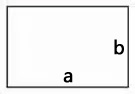### 长方形的周长 = (长 + 宽) × 2

$C = (a + b) \times 2$

### 长方形的面积 = 长 × 宽

$S = a \times b$

## 正方形：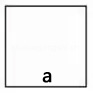### 正方形的周长 = 边长 × 4

$C = 4 \times a$

### 正方形的面积 = 边长 × 边长

$S = a \times a$

## 三角形：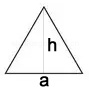### 三角形的面积 = 底 × 高 ÷ 2

$S = \frac{a \times h}{2}$

## 平行四边形：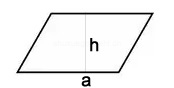### 平行四边形的面积 = 底 × 高

$S = a \times h$

## 梯形：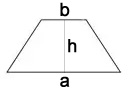### 梯形的面积 = (上底 + 下底) × 高 ÷ 2

$S = \frac{(a+b) \times h}{2}$

## 圆形：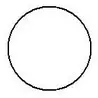### 直径 = 半径 × 2

$d=2r$

### 半径 = 直径 ÷ 2

$r=\frac{d}{2}$

### 圆的周长 = 圆周率 × 直径 = 圆周率 × 半径 × 2

$c=\pi d = 2 \pi r$

### 圆的面积 = 圆周率 × 半径 × 半径

$S = \pi r^2$

## 体积：

### 长方体：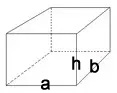• 长方体的体积 = 长 × 宽 × 高

$V = a\times b \times h$

• 长方体(或正方体)的体积 = 底面积 × 高

$V = a \times b \times h$

### 正方体：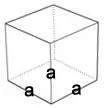• 正方体的体积 = 棱长 × 棱长 × 棱长

$V = a \times a \times a$

### 圆体：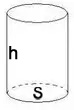• 圆柱的体积： 圆柱的体积等于底面积乘高

$V = S \times h$

### 圆锥：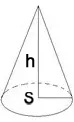• 圆锥的体积 = (底面积 × 高）÷ 3

$V = \frac{S \times h }{3}$

## 表面积：

1. 圆柱的表(侧)面积：圆柱的表(侧)面积等于底面的周长乘高

$S = c\times h=\pi \times d \times h=2\pi \times r \times h$

1. 圆柱的表面积：圆柱的表面积等于底面的周长乘高再加上两头的圆的面积。

$S = c \times h + 2S' = c \times h + 2\pi r^2$

## 分数：

1. 分数的加、减法则：
同分母的分数相加减，只把分子相加减，分母不变。
异分母的分数相加减，先通分，然后再加减。

2. 分数的乘法则：
用分子的积做分子，用分母的积做分母。

3. 分数的除法则：
除以一个数等于乘以这个数的倒数。